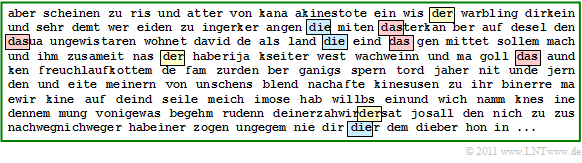# Aufgabe 1.8: Synthetisch erzeugte Texte

The former practical course attempt  Value Discrete Information Theory  by Günter Söder at the Chair of Communications Engineering at the TU Munich uses the Windows programme  WDIT.  The links given here lead to the PDF version of the practical course instructions or to the ZIP version of the programme.

With this programme

• from a given text file  "TEMPLATE"  one can determine the frequencies of letter triplets such as  "aaa",  "aab", ... ,  "xyz", ...  and save them in an auxiliary file,
• then create a file  "SYNTHESIS"  whereby the new character is generated from the last two characters and the stored triple frequencies.

Starting with the German and English Bible translations, we have thus synthesised two files, which are indicated in the diagram:

• die  $\text{File 1}$  (red border),
• die  $\text{File 2}$  (green border)

It is not indicated which file comes from which template.  Determining this is your first task.

The two templates are based on the natural alphabet  $(26$ letters$)$  and the space  ("LZ")   ⇒   $M = 27$.  In the German Bible, the umlauts have been replaced, for example "ä"   ⇒   "ae".

$\text{File 1}$  has the following characteristics:

• The most frequent characters are "LZ" with  $19.8\%$, followed by "e" with  $10.2\%$  and "a" with  $8.5\%$.
• After "LZ" (space), "t" occurs most frequently with  $17.8\%$ .
• Before a space, "d" is most likely.
• The entropy approximations in each case with the unit "bit/character" were determined as follows:
$$H_0 = 4.76\hspace{0.05cm},\hspace{0.2cm} H_1 = 4.00\hspace{0.05cm},\hspace{0.2cm} H_2 = 3.54\hspace{0.05cm},\hspace{0.2cm} H_3 = 3.11\hspace{0.05cm},\hspace{0.2cm} H_4 = 2.81\hspace{0.05cm}.$$

In contrast, the analysis of  $\text{file 2}$:

• The most frequent characters are "LZ" with  $17.6\%$  followed by "e" with  $14.4\%$  and "n" with  $8.9\%$.
• After "LZ", "d" is the most likely  $(15.1\%)$  followed by "s" with  $10.8\%$.
• After "LZ" and "d", the vowels "e"  $(48.3\%)$,  "i" $(23\%)$  and "a"  $(20.2\%)$  are dominant.
• The entropy approximations differ only slightly from those of  $\text{file 1}$.
• For larger  $k$–values, these are slightly larger, for example  $H_3 = 3.17$  instead of  $H_3 = 3.11$.

Hints:

### Questions

1

Which templates were used for the text synthesis shown here??

 Die  $\text{File 1}$  (red) is based on an English template. Die  $\text{File 1}$  (red) is based on a German template.

2

Compare the mean word lengths of  $\text{File 1}$  and  $\text{File 2}$ .

 The words of the "English" file are longer on average. The words of the "German" file are longer on average.

3

Which statements apply to the entropy approximations?

 "TEMPLATE"  and  "SYNTHESIS"  provide a nearly equal  $H_1$. "TEMPLATE"  and  "SYNTHESIS"  provide a nearly equal  $H_2$. "TEMPLATE"  and  "SYNTHESIS"  provide a nearly equal  $H_3$. "TEMPLATE"  and  "SYNTHESIS"  provide a nearly equal  $H_4$.

4

Which statements are true for the "English" text?

 Most words begin with  "t". Most words end with  "t".

5

Which statements could be true for German texts?

 After  "de"  ist  "r"  is most likely. After  "da"  ist  "s"  is most likely. After  "di"  ist  "e"  is most likely.

### Solution

#### Solution

(1)  The correct solution is suggestion 1.

• In  $\text{file 1}$  you can recognise many English words, in  $\text{file 2}$  many German words.
• Neither text makes sense.

(2)  Correct is suggestions 2. The estimations of Shannon and Küpfmüller confirm our result:

• The probability of a blank character in  $\text{file 1}$  (English)  $19.8\%$.
• So on average every  $1/0.198 = 5.05$–th character is a space.
• The average word length is therefore
$$L_{\rm M} = \frac{1}{0.198}-1 \approx 4.05\,{\rm characters}\hspace{0.05cm}.$$
• Correspondingly, for  $\text{file 2}$  (German):
$$L_{\rm M} = \frac{1}{0.176}-1 \approx 4.68\,{\rm characters}\hspace{0.05cm}.$$

(3)  The first three statements are correct, but not statement  (4):

• To determine the entropy approximation  $H_k$  ,  $k$–tuples must be evaluated, for example, for  $k = 3$  the triples   "aaa",  "aab",   ....
• According to the generation rule "New character depends on the two predecessors",  $H_1$,  $H_2$  and  $H_3$  of  "TEMPLATE"  and  "SYNTHESIS"  will match, but only approximately due to the finite file length.
• In contrast, the  $H_4$& approximations differ more strongly because the third predecessor is not taken into account during generation.
• It is only known that  "SYNTHESIS"  $H_4 < H_3$  must also apply with regard to

(4)  Only statement 1 is correct here:

• Nach einem Leerzeichen (Wortanfang) folgt "t" mit  $17.8\%$, während am Wortende (vor einem Leerzeichen) "t" nur mit der Häufigkeit  $<8.3\%$  auftritt.
• Insgesamt beträgt die Auftrittswahrscheinlichkeit von "t" über alle Positionen im Wort gemittelt  $7.4\%$.
• Als dritter Buchstaben nach Leerzeichen und "t" folgt "h" mit fast  $82\%$  und nach "th" ist "e" mit  $62%$  am wahrscheinlichsten.
• Das lässt daraus schließen, dass "the" in einem englischen Text überdurchschnittlich oft vorkommt und damit auch in der synthetischen  $\text{Datei 1}$, wie folgende Grafik zeigt. Aber nicht bei allen Markierungen tritt "the" isoliert auf   ⇒   direkt vorher und nachher ein Leerzeichen.

(5)  Alle Aussagen treffen zu:

• Nach "de" ist tatsächlich "r" am wahrscheinlichsten  $(32.8\%)$,  gefolgt von "n" $(28.5\%)$,  "s"  $(9.3\%)$  und "m"  $(9.7\%)$.
• Dafür verantwortlich könnten  "der",  "den",  "des"  und  "dem"  sein.Auftreten von "der", "die" und "das" im deutschen Text
• Nach "da" folgt "s" mit größter Wahrscheinlichkeit:   $48.2\%$.
• Nach "di" folgt "e" mit größter Wahrscheinlichkeit:   $78.7\%$.

Die Grafik zeigt die  $\text{Datei 2}$  mit allen "der", "die" und "das".